``````## Paul E. Johnson
## 2014-09-18

## Here's a puzzle I presented to our group this week.

## Generate data like so

set.seed(234234)
dat <- data.frame(x1 = rnorm(100, m = 50, sd = 40), x2 = gl(4, 25,
labels = c("l", "m", "h", "s")), x3 = rgamma(100, 1, 2))
dat\$y <- rpois(100, lambda = exp(0.005 + 0.014 * dat\$x1 - 0.1 * dat\$x3))
m1 <- glm(y ~ x1 + x2 + x3, data = dat, family = "poisson")
m2 <- glm(y ~ x1, data = dat, family = "poisson")

## Exercises

## 1. Make a plot demonstrating the relationship between x1 and y,
## including the predicted values from both m1 and m2.

## 2. Suppose you want to plot the predicted value of y, for each
## categorical value of x2.  What would you do?

## 2A. What is the difference between

predict(m1)
``````
``````##        1        2        3        4        5        6        7        8
##  0.78761  0.57589  0.93523  0.57266  0.76530  1.28713  0.93793  0.98241
##        9       10       11       12       13       14       15       16
##  1.31750  0.68068  1.43293  0.73529  1.10346  0.72862  0.56668  0.94110
##       17       18       19       20       21       22       23       24
##  1.94896  0.04967  0.96010  0.19668  1.41698  1.35944  0.04705  0.42981
##       25       26       27       28       29       30       31       32
## -0.22183  1.34179  0.33170 -0.01971  0.39195  0.99532  0.54241  0.48098
##       33       34       35       36       37       38       39       40
##  0.73244  0.50376  0.30670  0.64904  1.21870 -0.16999  1.12084  1.01777
##       41       42       43       44       45       46       47       48
##  0.90110  1.19787  0.81861  0.21943  1.06838  0.07575  0.76713  2.13945
##       49       50       51       52       53       54       55       56
##  0.15473  0.74920 -0.52417 -0.31611  0.68070 -0.02657  1.10092  0.02224
##       57       58       59       60       61       62       63       64
##  1.01728  0.32843 -0.33360  1.40265  0.01630  0.40897  0.22931  0.51323
##       65       66       67       68       69       70       71       72
##  0.32363  0.78681  0.29791  0.85760  0.64160 -0.13928 -0.12438  0.25258
##       73       74       75       76       77       78       79       80
##  0.59972  0.85563 -0.08751  1.02280  0.46102  0.07333  0.34797 -0.01053
##       81       82       83       84       85       86       87       88
## -0.01263  1.20155 -0.50061  0.57326  1.03216  0.26116 -0.45316  0.63864
##       89       90       91       92       93       94       95       96
##  0.56602  0.72965  0.38354  0.75460  0.42533  0.39742  0.97632  0.52491
##       97       98       99      100
##  0.50616  0.78810  0.83690  0.62237
``````
``````## and

predict(m1, type = "response")
``````
``````##      1      2      3      4      5      6      7      8      9     10
## 2.1981 1.7787 2.5478 1.7730 2.1496 3.6224 2.5547 2.6709 3.7341 1.9752
##     11     12     13     14     15     16     17     18     19     20
## 4.1909 2.0861 3.0146 2.0722 1.7624 2.5628 7.0214 1.0509 2.6120 1.2174
##     21     22     23     24     25     26     27     28     29     30
## 4.1246 3.8940 1.0482 1.5370 0.8010 3.8259 1.3933 0.9805 1.4799 2.7056
##     31     32     33     34     35     36     37     38     39     40
## 1.7202 1.6177 2.0802 1.6549 1.3589 1.9137 3.3828 0.8437 3.0674 2.7670
##     41     42     43     44     45     46     47     48     49     50
## 2.4623 3.3131 2.2673 1.2454 2.9107 1.0787 2.1536 8.4948 1.1673 2.1153
##     51     52     53     54     55     56     57     58     59     60
## 0.5920 0.7290 1.9753 0.9738 3.0069 1.0225 2.7657 1.3888 0.7163 4.0660
##     61     62     63     64     65     66     67     68     69     70
## 1.0164 1.5053 1.2577 1.6707 1.3821 2.1964 1.3470 2.3575 1.8995 0.8700
##     71     72     73     74     75     76     77     78     79     80
## 0.8830 1.2873 1.8216 2.3529 0.9162 2.7810 1.5857 1.0761 1.4162 0.9895
##     81     82     83     84     85     86     87     88     89     90
## 0.9874 3.3253 0.6062 1.7740 2.8071 1.2984 0.6356 1.8939 1.7612 2.0744
##     91     92     93     94     95     96     97     98     99    100
## 1.4675 2.1268 1.5301 1.4880 2.6547 1.6903 1.6589 2.1992 2.3092 1.8633
``````
``````## 2B. What is the difference between

predict(m1, type = "response")
``````
``````##      1      2      3      4      5      6      7      8      9     10
## 2.1981 1.7787 2.5478 1.7730 2.1496 3.6224 2.5547 2.6709 3.7341 1.9752
##     11     12     13     14     15     16     17     18     19     20
## 4.1909 2.0861 3.0146 2.0722 1.7624 2.5628 7.0214 1.0509 2.6120 1.2174
##     21     22     23     24     25     26     27     28     29     30
## 4.1246 3.8940 1.0482 1.5370 0.8010 3.8259 1.3933 0.9805 1.4799 2.7056
##     31     32     33     34     35     36     37     38     39     40
## 1.7202 1.6177 2.0802 1.6549 1.3589 1.9137 3.3828 0.8437 3.0674 2.7670
##     41     42     43     44     45     46     47     48     49     50
## 2.4623 3.3131 2.2673 1.2454 2.9107 1.0787 2.1536 8.4948 1.1673 2.1153
##     51     52     53     54     55     56     57     58     59     60
## 0.5920 0.7290 1.9753 0.9738 3.0069 1.0225 2.7657 1.3888 0.7163 4.0660
##     61     62     63     64     65     66     67     68     69     70
## 1.0164 1.5053 1.2577 1.6707 1.3821 2.1964 1.3470 2.3575 1.8995 0.8700
##     71     72     73     74     75     76     77     78     79     80
## 0.8830 1.2873 1.8216 2.3529 0.9162 2.7810 1.5857 1.0761 1.4162 0.9895
##     81     82     83     84     85     86     87     88     89     90
## 0.9874 3.3253 0.6062 1.7740 2.8071 1.2984 0.6356 1.8939 1.7612 2.0744
##     91     92     93     94     95     96     97     98     99    100
## 1.4675 2.1268 1.5301 1.4880 2.6547 1.6903 1.6589 2.1992 2.3092 1.8633
``````
``````## and

predict(m2, type = "response")
``````
``````##      1      2      3      4      5      6      7      8      9     10
## 1.7928 1.2972 2.1088 1.4514 1.7367 2.7586 2.0980 2.0982 3.1104 1.3746
##     11     12     13     14     15     16     17     18     19     20
## 3.4362 1.6375 2.3996 1.5612 1.3870 2.0594 5.8368 0.8409 2.1651 0.9724
##     21     22     23     24     25     26     27     28     29     30
## 3.5021 3.2569 0.8194 1.1892 0.6045 3.8685 1.3651 0.9339 1.1128 2.6954
##     31     32     33     34     35     36     37     38     39     40
## 1.5926 1.6230 2.0055 1.6702 1.3396 1.9145 3.5025 0.8329 3.1139 2.6333
##     41     42     43     44     45     46     47     48     49     50
## 2.4356 3.4123 2.1988 1.2007 2.8922 1.0642 2.0026 7.1653 1.1177 1.9934
##     51     52     53     54     55     56     57     58     59     60
## 0.7139 0.8753 2.5741 1.2623 3.5495 1.1535 3.6667 1.8268 0.8701 5.5313
##     61     62     63     64     65     66     67     68     69     70
## 1.3202 1.8811 1.6449 2.0411 1.6702 2.6356 1.6910 3.1345 2.4700 1.1113
##     71     72     73     74     75     76     77     78     79     80
## 1.1154 1.5746 2.3968 2.8858 1.1378 2.8355 1.5522 1.1793 1.5106 1.0968
##     81     82     83     84     85     86     87     88     89     90
## 1.0317 3.8087 0.6507 2.0004 2.9785 1.3665 0.6506 1.9637 1.9971 2.3624
##     91     92     93     94     95     96     97     98     99    100
## 1.6549 2.4183 1.6263 1.6550 2.9992 1.8874 1.8589 2.4455 2.6275 1.9267
``````
``````## 3. What's wrong with this approach?

pred1 <- predict(m1)
plot(pred1 ~ dat\$x1, type = "l")
````````````## 4. What's wrong with this approach?

dat <- dat[order(dat\$x1), ]
m1 <- glm(y ~ x1 + x2 + x3, data = dat, family = "poisson")
pred2 <- predict(m1)
plot(y ~ x1, data = dat)
lines(pred2 ~ x1, data = dat)

## 5. What's wrong with this approach?

library(rockchalk)
````````````nd <- newdata(m1, predVals = c("x1"), n = 20)
nd\$pred <- predict(m1, nd)
plot(y ~ x1, data = dat)
lines(pred ~ x1, data = nd)
````````````## 6. Can you make a pleasant looking regression table for m1 and m2?
## If not, try R packages that can like memisc, texreg, stargazer,
## apsrtable, rockchalk, or ...

## 7. More advanced: what's the problem with getting confidence
## intervals on that predicted value line?

## Can you explain why I feel like a cheater when I wrote this function?

plotSlopes(m1, plotx = x1, interval = "conf")
``````
``````## rockchalk:::predCI: model's predict method does not return an interval. We will improvize with a Wald type approximation to the confidence interval
``````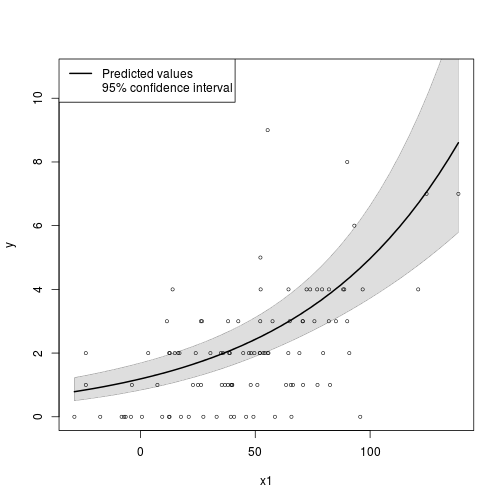``````## If you did not ever try to work with predicted value plots, I
## eagerly suggest you try it.

## 1. Make a plot demonstrating the relationship between x1 and y,
## including the predicted values from both m1 and m2.

## m2 is easy. Do that first
m2nd <- newdata(m2, predVals = list(x1 = "quantile"), n = 30)
m2nd\$fit <- predict(m2, newdata = m2nd, type = "response")

plot(y ~ x1, data = dat, col = "gray70")
lines(fit ~ x1, data = m2nd)
``````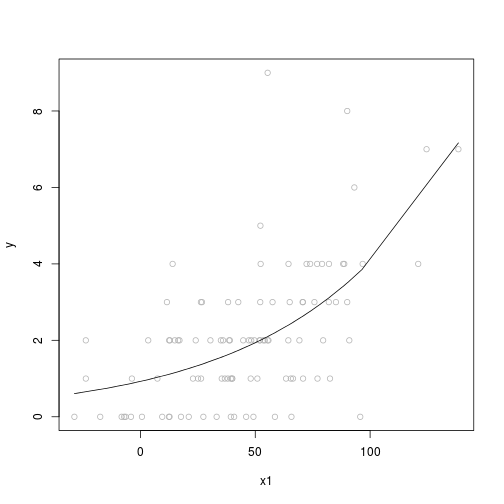``````## Perhaps it is more clear to look at it like this
plot(y ~ x1, data = dat, col = "gray70")
lines(fit ~ x1, data = m2nd, type = "b")
title("30 quantile-spaced values of x1")
``````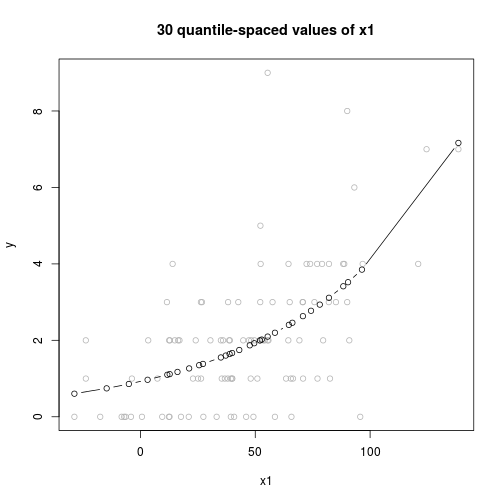``````## Obvious problem: What values of x1 should be used? 30 quantiles?

m2nd2 <- newdata(m2, predVals = list(x1 = "seq"), n = 30)
m2nd2\$fit <- predict(m2, newdata = m2nd2, type = "response")
plot(y ~ x1, data = dat, col = "gray70")
lines(fit ~ x1, data = m2nd2, type = "b")
title("Evenly spaced values of x1")

## Obvious problem. What values are to be supposed for x2 and x3?
m1nd <- newdata(m1, predVals = list(x1 = "quantile", x2 = levels(dat\$x2)), n = 20)
m1nd
``````
``````##         x1 x2     x3
## 1  -28.737  l 0.4613
## 2   -7.123  l 0.4613
## 3    3.132  l 0.4613
## 4   12.508  l 0.4613
## 5   16.146  l 0.4613
## 6   23.781  l 0.4613
## 7   27.231  l 0.4613
## 8   35.789  l 0.4613
## 9   38.822  l 0.4613
## 10  40.438  l 0.4613
## 11  47.617  l 0.4613
## 12  51.380  l 0.4613
## 13  52.823  l 0.4613
## 14  56.367  l 0.4613
## 15  64.634  l 0.4613
## 16  69.572  l 0.4613
## 17  74.276  l 0.4613
## 18  79.927  l 0.4613
## 19  88.331  l 0.4613
## 20  93.273  l 0.4613
## 21 138.443  l 0.4613
## 22 -28.737  m 0.4613
## 23  -7.123  m 0.4613
## 24   3.132  m 0.4613
## 25  12.508  m 0.4613
## 26  16.146  m 0.4613
## 27  23.781  m 0.4613
## 28  27.231  m 0.4613
## 29  35.789  m 0.4613
## 30  38.822  m 0.4613
## 31  40.438  m 0.4613
## 32  47.617  m 0.4613
## 33  51.380  m 0.4613
## 34  52.823  m 0.4613
## 35  56.367  m 0.4613
## 36  64.634  m 0.4613
## 37  69.572  m 0.4613
## 38  74.276  m 0.4613
## 39  79.927  m 0.4613
## 40  88.331  m 0.4613
## 41  93.273  m 0.4613
## 42 138.443  m 0.4613
## 43 -28.737  h 0.4613
## 44  -7.123  h 0.4613
## 45   3.132  h 0.4613
## 46  12.508  h 0.4613
## 47  16.146  h 0.4613
## 48  23.781  h 0.4613
## 49  27.231  h 0.4613
## 50  35.789  h 0.4613
## 51  38.822  h 0.4613
## 52  40.438  h 0.4613
## 53  47.617  h 0.4613
## 54  51.380  h 0.4613
## 55  52.823  h 0.4613
## 56  56.367  h 0.4613
## 57  64.634  h 0.4613
## 58  69.572  h 0.4613
## 59  74.276  h 0.4613
## 60  79.927  h 0.4613
## 61  88.331  h 0.4613
## 62  93.273  h 0.4613
## 63 138.443  h 0.4613
## 64 -28.737  s 0.4613
## 65  -7.123  s 0.4613
## 66   3.132  s 0.4613
## 67  12.508  s 0.4613
## 68  16.146  s 0.4613
## 69  23.781  s 0.4613
## 70  27.231  s 0.4613
## 71  35.789  s 0.4613
## 72  38.822  s 0.4613
## 73  40.438  s 0.4613
## 74  47.617  s 0.4613
## 75  51.380  s 0.4613
## 76  52.823  s 0.4613
## 77  56.367  s 0.4613
## 78  64.634  s 0.4613
## 79  69.572  s 0.4613
## 80  74.276  s 0.4613
## 81  79.927  s 0.4613
## 82  88.331  s 0.4613
## 83  93.273  s 0.4613
## 84 138.443  s 0.4613
``````
``````## See the problem?:
m1nd\$fit <- predict(m1, newdata = m1nd, type = "response")
text(m1nd\$x1, m1nd\$fit, labels = as.character(m1nd\$x2))
``````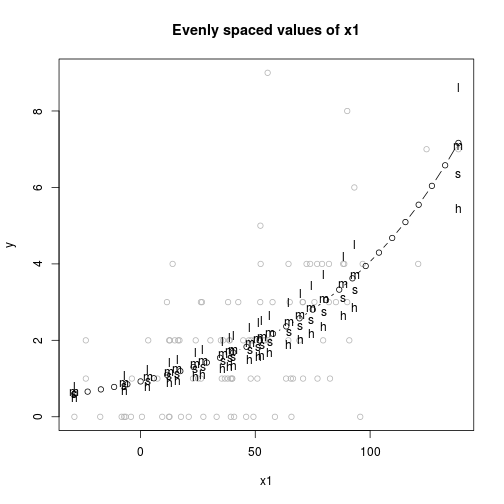``````plot(y ~ x1, data = dat, col = "gray70")
mycol <- gray.colors(4, start = 0.3, end = 0.6)
x2levels <- levels(m1nd\$x2)
for(i in seq_along(x2levels)){
lines(fit ~ x1, data = m1nd[m1nd\$x2 %in% x2levels[i], ], lty = i + 1, col = mycol[i], lwd = 2)
}
lines(fit ~ x1, data = m2nd)
legend("topleft", legend = c("Model 2", paste("Model 1: x2 =", x2levels)), col = c(1, mycol), lty = 1:5, lwd = 2)
``````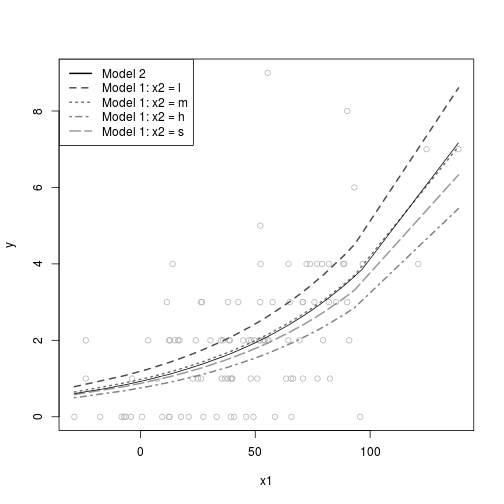``````## The rockchalk package has a short-cut function for most of this work, but it won't integrate
## m1 and m2 for us.

## ## 4. What's wrong with this approach?

## dat <- dat[order(dat\$x1), ]
## m1 <- glm(y ~ x1 + x2 + x3, data = dat, family = "poisson")
## pred2 <- predict(m1)
## plot(y ~ x1, data = dat)
## lines(pred2 ~ x1, data = dat)

## Predicted values are calculated for each row in the data. The
## plot looks/is wrong because we have not controlled for x2 and x3
## in the calculation of the predicted values for plotting.

## ## 5. What's wrong with this approach?

## library(rockchalk)
## nd <- newdata(m1, predVals = c("x1"), n = 20)
## nd\$pred <- predict(m1, nd)
## plot(y ~ x1, data = dat)
## lines(pred ~ x1, data = nd)

## That's almost right. Inspect nd, see it has adjusted x2 and x3.
## However, the predict statement needs type = "response"

library(rockchalk)
nd <- newdata(m1, predVals = c("x1"), n = 20)
nd\$fit <- predict(m1, nd, type = "response")
plot(y ~ x1, data = dat)
lines(fit ~ x1, data = nd)
````````````## do you want various predictive lines?

plotCurves(m1, plotx = "x1", modx = "x2")
``````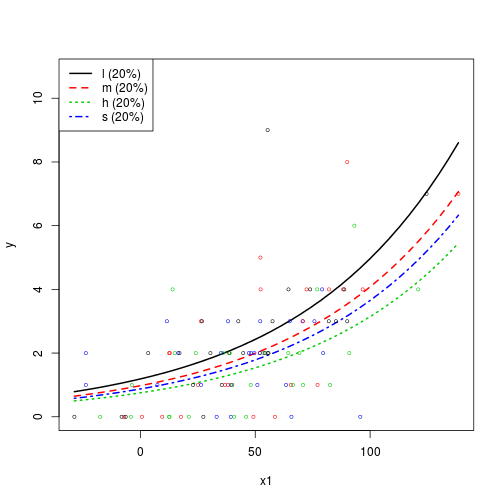``````##
##
## Now, I want to take you on 2 detours.

## Detour 1. Plot predictions by quantile.

## Lets just ask for 5 values

nd2 <- newdata(m1, predVals = list(x1 = "quantile", x2 = "l"), n = 5)
nd2\$fit <- predict(m1, nd2, type = "response")

par(mfcol = c(2,1))

plot(y ~ x1, data = dat, col = "gray70")
lines(fit ~ x1, data = nd2, type = "b")

nd2\$q1 <- c("min", "25%", "median", "75%", "max")
nd2\$q2 <- 1:5

plot(fit ~ q2, data = nd2, type = "b", xlab = "x1 quantiles", axes = FALSE)
axis(2)
axis(1, at = nd2\$q2, labels = nd2\$q1)
box(bty = "L")
``````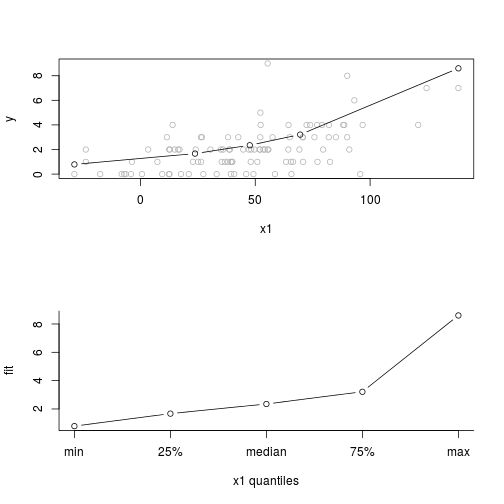``````par(par.orig)

## If x1's distribution is less symmetric,the problem is exaggerated

set.seed(234234)
dat2 <- data.frame(x1 = 1.5 *  rgamma(100, shape = 2, scale = 18.3),
x2 = gl(4, 25, labels = c("l", "m", "h", "s")),
x3 = rgamma(100, 1, 2))
dat2\$y <- rpois(100, lambda = exp(0.005 + 0.014 * dat2\$x1 - 0.1 * dat2\$x3))

summary(dat)
``````
``````##        x1        x2           x3               y
##  Min.   :-28.7   l:25   Min.   :0.0046   Min.   :0.00
##  1st Qu.: 23.8   m:25   1st Qu.:0.1284   1st Qu.:1.00
##  Median : 47.6   h:25   Median :0.3293   Median :2.00
##  Mean   : 46.0   s:25   Mean   :0.4613   Mean   :2.06
##  3rd Qu.: 69.6          3rd Qu.:0.6377   3rd Qu.:3.00
##  Max.   :138.4          Max.   :2.8342   Max.   :9.00
``````
``````summary(dat2)
``````
``````##        x1         x2           x3              y
##  Min.   :  2.89   l:25   Min.   :0.002   Min.   : 0.00
##  1st Qu.: 27.75   m:25   1st Qu.:0.149   1st Qu.: 1.00
##  Median : 47.99   h:25   Median :0.354   Median : 2.00
##  Mean   : 54.72   s:25   Mean   :0.461   Mean   : 2.71
##  3rd Qu.: 69.98          3rd Qu.:0.703   3rd Qu.: 3.00
##  Max.   :174.79          Max.   :2.306   Max.   :16.00
``````
``````hist(dat2\$x1)
````````````m1.2 <- glm(y ~ x1 + x2 + x3, data = dat2, family = "poisson")

nd3 <- newdata(m1.2, predVals = list(x1 = "quantile", x2 = "l"), n = 5)
nd3\$fit <- predict(m1.2, nd3, type = "response")

par(mfcol = c(2,1))

plot(y ~ x1, data = dat2, col = "gray70")
lines(fit ~ x1, data = nd3, type = "b")

nd3\$q1 <- c("min", "25%", "median", "75%", "max")
nd3\$q2 <- seq(min(nd3\$x1), max(nd3\$x1), length.out = 5)

plot(fit ~ q2, data = nd3, type = "b", xlab = "x1 quantiles", axes = FALSE, col = "gray70")
axis(2)
axis(1, at = nd3\$q2, labels = nd3\$q1)
box(bty = "L")
lines(fit ~ q2, data = nd3, type = "b")
``````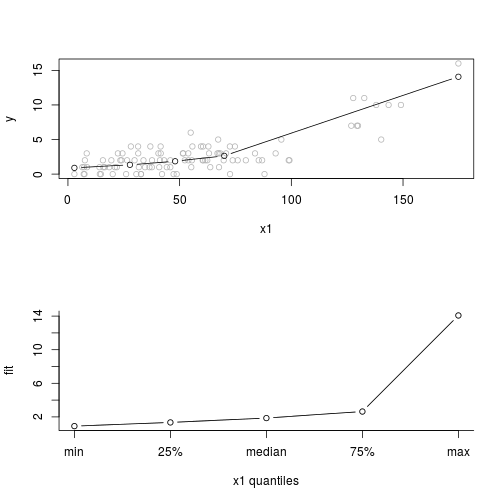``````par(par.orig)

## What are the dangers here?

## Why might one want to do this?

## Detour 2. What if the data has only 2 or 3 unique values of a predictor?

set.seed(234234)
dat <- data.frame(x1 = rnorm(100, m = 50, sd = 40),
x2 = gl(4, 25, labels = c("l", "m", "h", "s")),
x3 = rgamma(100, 1, 2))
dat\$x1f <- cut(dat\$x1, c(-100, 50, 80, 200), labels = c("low", "med", "high"))
dat\$x1n <- as.numeric(dat\$x1f)
dat\$y <- rpois(100, lambda = exp(0.005 + 0.014 * dat\$x1 - 0.1 * dat\$x3))

m1 <- glm(y ~ x1n + x2 + x3, data = dat, family = "poisson")
summary(m1)
``````
``````##
## Call:
## glm(formula = y ~ x1n + x2 + x3, family = "poisson", data = dat)
##
## Deviance Residuals:
##     Min       1Q   Median       3Q      Max
## -2.7697  -0.9699  -0.0865   0.5910   3.0815
##
## Coefficients:
##             Estimate Std. Error z value Pr(>|z|)
## (Intercept)  -0.2765     0.2315   -1.19    0.232
## x1n           0.5952     0.0888    6.70    2e-11 ***
## x2m          -0.1629     0.1841   -0.88    0.376
## x2h          -0.4082     0.2019   -2.02    0.043 *
## x2s          -0.1977     0.1985   -1.00    0.319
## x3            0.2404     0.1273    1.89    0.059 .
## ---
## Signif. codes:  0 '***' 0.001 '**' 0.01 '*' 0.05 '.' 0.1 ' ' 1
##
## (Dispersion parameter for poisson family taken to be 1)
##
##     Null deviance: 167.37  on 99  degrees of freedom
## Residual deviance: 114.33  on 94  degrees of freedom
## AIC: 340.7
##
## Number of Fisher Scoring iterations: 5
``````
``````nd4 <- newdata(m1, predVals = list(x1n = "quantile", x2 = "l"), n = 5)
nd4\$fit <- predict(m1, newdata = nd4, type = "response")
nd4
``````
``````##   x1n x2     x3   fit
## 1   1  l 0.4613 1.537
## 2   2  l 0.4613 2.787
## 3   3  l 0.4613 5.053
``````
``````plot(fit ~ x1n, data = nd4, type = "b")
````````````## Question: Should newdata refuse to give back more possible values of
## x1n than there actually are?

## I'll fake it by manufacturing data points at 1.5 and 2.5
x1quant <- quantile(dat\$x1n)
nd5 <- expand.grid(x1n = seq(1, 3, length.out = 5), x2 = "l", x3 = mean(dat\$x3))
nd5\$fit <- predict(m1, newdata = nd5, type = "response")
nd5
``````
``````##   x1n x2     x3   fit
## 1 1.0  l 0.4613 1.537
## 2 1.5  l 0.4613 2.069
## 3 2.0  l 0.4613 2.787
## 4 2.5  l 0.4613 3.752
## 5 3.0  l 0.4613 5.053
``````
``````plot(fit ~ x1n, data = nd5, type = "b", xlab = "phony (?) x1 quantiles", axes = FALSE)
axis(2)
axis(1, at = nd5\$x1n, labels = nd5\$q1)
box(bty = "L")
````````````## ## 7. More advanced: what's the problem with getting confidence
## ## intervals on that predicted value line?

## ## Can you explain why I feel like a cheater when I wrote this function?

## plotSlopes(m1, plotx = x1, interval = "conf")

## ## If you did not ever try to work with predicted value plots, I
## ## eagerly suggest you try it.

## Those calculations rely on a very simplistic "Wald approximation"
## to calculate confidence intervals on predictions. These are also
## supported in SAS, but the literature is generally calling for more
## elaborate methods none of which are easily available.

## Withers, Christopher S. and Saralees Nadarah. 2012. Improved
## confidence regions based on Edgeworth expansions. Computational
## Statistics and Data Analysis 56: 4366-4380.

## "The number of papers proposing confidence regions is too many to
## cite. Confidence regions can be constructed using many different
## methods. Some of these involve: the exact distribution theory,
## asymptotic chi-square distributions, asymptotic normal distributions,
## record values, bootstrapping, empirical likelihood method, likelihood
## ratio statistics, Bayesian test statistics, sign based test
## statistics, rank based test statistics, differential geometric
## frameworks, jackknife, least square statistics, sequential methods,
## Monte Carlo likelihood methods, adaptive estimation methods, the M
## estimation theory, empirical Bayes, calibration methods, score
## statistics, quadratic approximations, subsampling methods, Gibbs
## sampling methods, smoothing methods (for example, polynomial splines,
## kernel type smoothing, local adaptive smoothing and Granulometric
## smoothing), Kolmogorov–Smirnov statistics, highest posterior density
## methods, R estimation methods, stochastic inequalities (for example,
## Hajek–Rényi type inequalities), multiple comparison methods, maximum
## modulus methods, minimum area confidence methods, U statistics, Rényi
## statistics and statistics based on other entropy measures, generalized
## p values, James–Stein type statistics, power divergence statistics,
## importance sampling methods, Uusipaikka’s method, genetic algorithms
## and uniform minimum variance unbiased statistics."

## King, Gary, Michael Tomz, and Jason Wittenberg. 2000. Making the Most
## of Statistical Analyses: Improving Interpretation and
## Presentation. American Journal of Political Science 44: 341–355

## Sun, J., Loader, C., & William P., M. (2000). Confidence bands in
## generalized linear models. The Annals of Statistics, 28(2),
## 429–460. doi:10.1214/aos/1016218225

## Xu, Jun and J. Scott Long (2005) Confidence intervals for predicted outcomes in
## regression models for categorical outcomes. Stata Journal 5(4): 537-559.

## 6. Can you make a pleasant looking regression table for m1 and m2?
## If not, try R packages that can like memisc, texreg, stargazer,
## apsrtable, rockchalk, or ...

varLabs <- c(x1 = "Flowers", x2l = "x2: low", x2m = "x2: medium",
x2h = "x2: high", x2s = "x2: super", x3 = "Nearly Forgot x3's super long label")
outreg(list("Model 1" = m1, "Model 2" = m2),  varLabels = varLabs, type = "html")
``````
``````##
##  We are launching a browser to view that html file, which we have temporarily
##  saved in  /tmp/RtmpFUk9T8/file10705954650b.html
##  You can copy that temp file, or create
##  one of your own with R's cat function.  Like this:
## myreg <-
## outreg(modelList = list(`Model 1` = m1, `Model 2` = m2), type = "html",
##     varLabels = varLabs)
##
##  cat(myreg, file = "reg.html")
##  Then open reg.html in a word processor or web browser.
``````